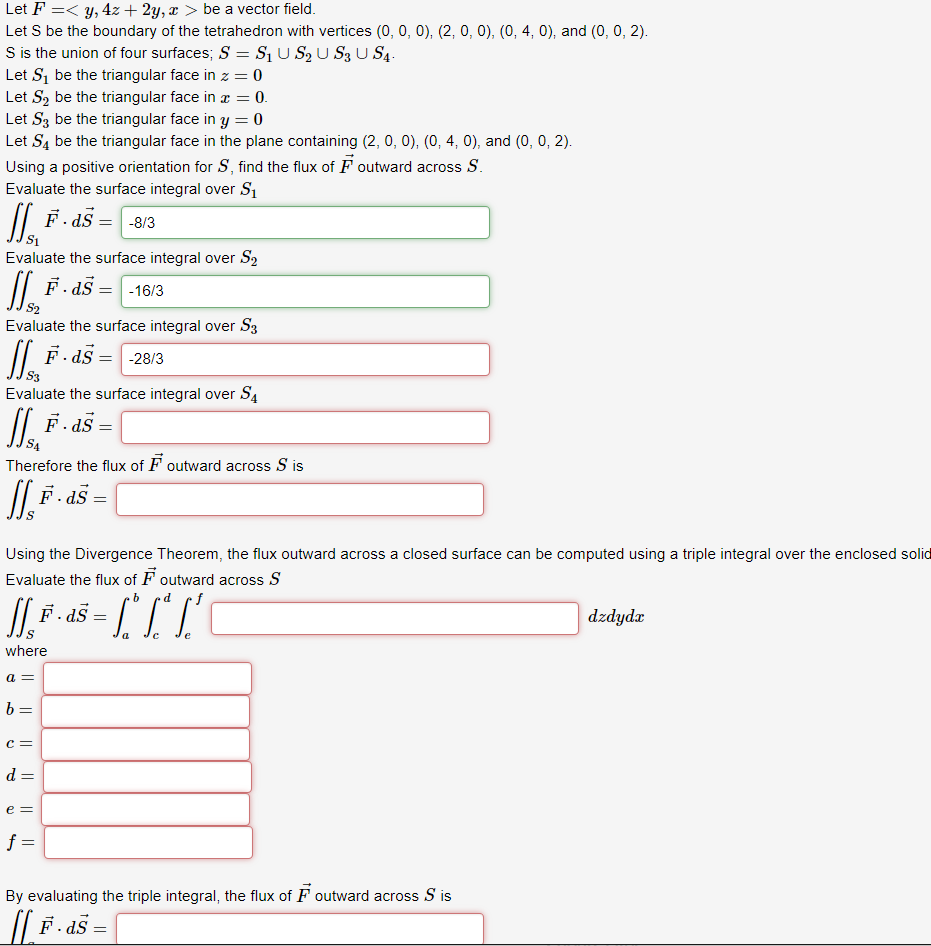# Solved: Let F Y, 4z2y, X > Be A Vector Field. Let S Be The Boundary Of The Tetrahedron With Vertices (0, 0, 0), (2, 0, 0), (0, 4, 0), And (0, 0, 2) S Is The Union Of Four Surfaces, S S1U S2 U S3 U S4

By |Let F y, 4z2y, x > be a vector field. Let S be the boundary of the tetrahedron with vertices (0, 0, 0), (2, 0, 0), (0, 4, 0), and (0, 0, 2) S is the union of four surfaces, S S1U S2 U S3 U S4 Let S1 be the triangular face in z= 0 Let S2 be the triangular face in x = 0 Let S3 be the triangular face in y 0 Let S4 be the triangular face in the plane containing (2, 0, 0), (0, 4, 0), and (0, 0, 2) Using a positive orientation for S, find the flux of F outward across S Evaluate the surface integral over S? FdS =-8/3 Evaluate the surface integral over S2 F dS -16/3 S2 Evaluate the surface integral over S3 F dS =-28/3 S3 Evaluate the surface integral over SA F. dS S4 Therefore the flux of F outward across S is F.dS = S Using the Divergence Theorem, the flux outward across a closed surface can be computed using a triple integral over the enclosed solic Evaluate the flux of F outward across S b d dzdydx S e: 0,7,17= where a= h C = d = ? — By evaluating the triple integral, the flux of F outward across S is